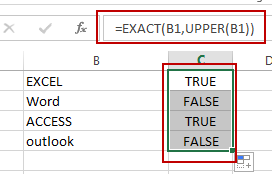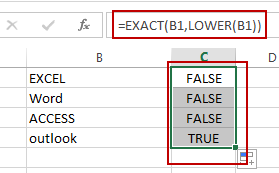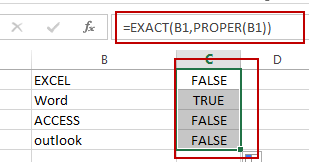# How to Check If a Cell is Uppercase, Lowercases or Capitalize in Excel

This post will guide you how to check if a cell is uppercase in Excel 2013/2016. How do I identify all letters in cells are lowercases or capitalize in Excel. How to change letters to uppercase, lowercases or capitalize first letter for all words in cells in Excel.

## Check If All Letters are Uppercase

If you want to check if all letters in cells are uppercase, you can use a formula based on the EXACT function and the UPPER function. Like this:

`=EXACT(B1,UPPER(B1))`

Type this formula into a blank cell and press Enter key to apply this formula. If All letters are uppercases, and it returns TRUE. Otherwise, it returns FALSE. Then drag the AutoFill Handle down to other cells to apply this formula.Let’ see how this formula works:

The UPPER function will convert all letters to uppercase. And then pass the result to EXACT function as its second argument. And the EXACT function will compare if two values are equal. If equal, returns TRUE. Otherwise, returns FALSE.

## Check If All Letters are Lowercase

If you need to identify all characters in cells are lowercase, you can use a formula based on the LOWER function and the EXACT function. Like this:

`=EXACT(B1,LOWER(B1))`

Type this formula into cell C1, and press Enter key to apply this formula. If all letters are lowercases, and it returns TRUE. Otherwise, it returns FALSE. Then drag the AutoFill Handle down to other cells to apply this formula.The LOWER function converts all letters to lowercase.

## Check If the First Letters are capitalized

If you want to check if the first character in each word is capitalized, you can use a formula based on the EXACT function and the PROPER function. Like this:

`=EXACT(B1,PROPER(B1))`

Type this formula into cell C1, and press Enter key to apply this formula. If all the first letters are capitalized, and it returns TRUE. Otherwise, it returns FALSE. Then drag the AutoFill Handle down to other cells to apply this formula.The PROPER function will capitalize the first letter in each word in Cell.

### Related Functions

• Excel UPPER function
The Excel UPPER function converts all characters in text string to uppercase.The UPPER function is a build-in function in Microsoft Excel and it is categorized as a Text Function.The syntax of the UPPER function is as below:= UPPER (text)…
• Excel LOWER function
The Excel LOWER function converts all characters in a text string to lowercase.The syntax of the LOWER function is as below:= LOWER  (text)…
• Excel Proper Function
The Excel PROPER function capitalizes the first character in each word of a text string and set other characters to lowercase. The syntax of the PROPER function is as below:= PROPER  (text)…
• Excel EXACT function
The Excel EXACT function compares if two text strings are the same and returns TRUE if they are the same, Or, it will return FALSE.The syntax of the EXACT function is as below:= EXACT (text1,text2)…